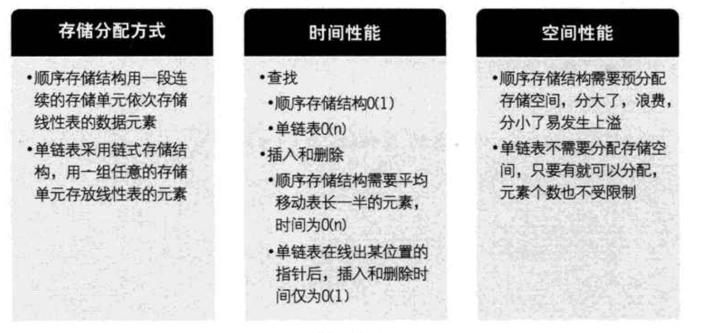## 链表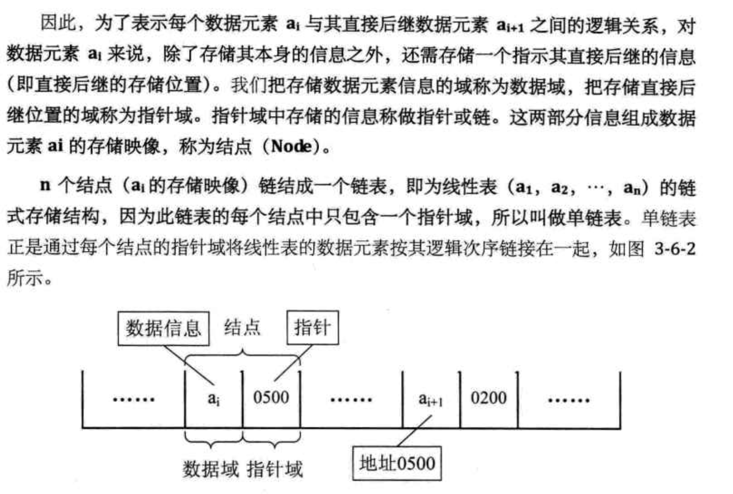### 一些概念

• 节点： 包含两个部分，数据信息和下一个节点的指针
• 头指针： 链表中第一个节点的存储位置。链表最后一个节点的指针为空，通常用`NULL`表示
• 头节点： 为了方便对链表进行操作，会在第一个节点前附设一个节点，称为头节点。头节点的数据域可以不存放任何信息，也可以存放链表的长度，指针域指向第一个节点。

#### 头指针与头结点的区别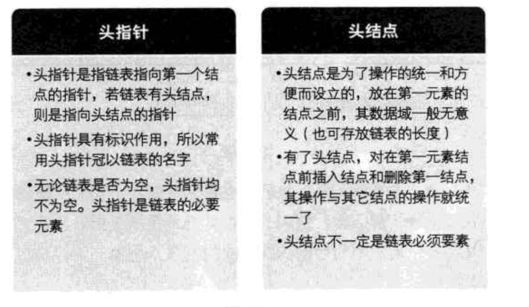#### 示意图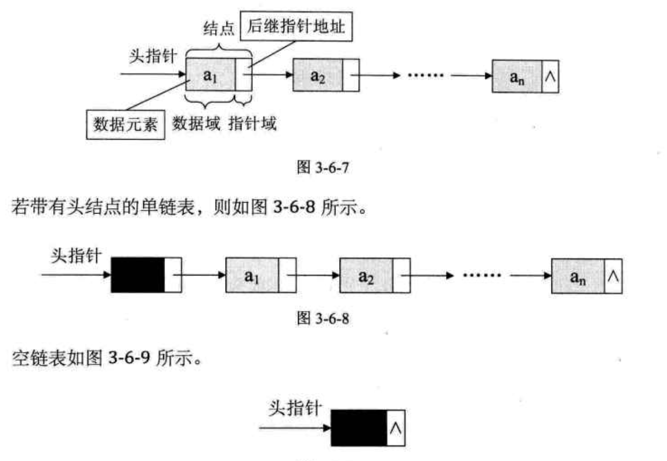### 单链表操作

#### 插入操作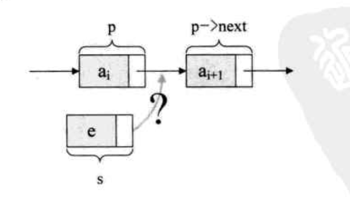``````//注意先后顺序不要写反了
s->next = p->next
p->next = s
``````

``````const list = new LinkedList()
list.insert(index, elem)
``````
1. 初始化 j 从 1 开始，声明一个节点 p 指向链表的第一个节点，如果有头节点，就是指向头节点
2. `j<index`时，就遍历链表，让 p 的指针往后移，不断指向下一个节点，j 累加
3. 若到链表末尾 p 为空，则说明第 index 个元素不存在
4. 否则查找成功，在系统中生成一个空节点 s
5. 将数据元素 elem 赋值给 s
6. 单链表插入的核心语句：`s->next = p->next; p->next = s`
7. 链表长度+1，返回成功
``````//声明一个单链表结点
class Node {
constructor(data) {
this.data = data //数据域
this.next = null //指针域
}
}

/**
* 链表数据结构
*/
constructor() {
this.length = 0 //存放链表的长度
}

/**
* 单链表的插入，在节点之前插入
* @param {*} index 第几个节点
* @param {*} elem  插入的数据
* @returns
*/
insert(index, elem) {
const j = 1,
//找到第index个节点
while (p && j < index) {
p = p.next
++j
}
//如果没找到返回false
if (!p || j > index) {
return false
}
//声明一个要插入的节点
const s = new Node(elem)
//插入核心逻辑
s.next = p.next
p.next = s
//链表长度加1
++this.length
//返回链表本身，使其支持链式操作
return this
}
}

``````

#### 删除操作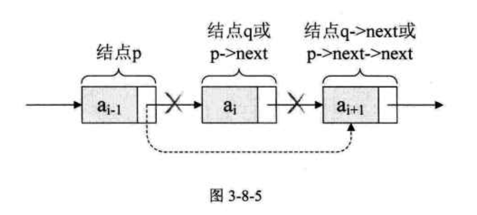``````//通过p->next找到要删除的节点
q = p->next
//指针指向 q->next
p->next = q->next
``````

``````const list = new LinkedList()
list.delete(index)
``````
1. 跟插入一样，先找到 index 的前一个节点，判断边界情况
2. 找到后，使用删除的核心逻辑`q = p->next, p->next = q->next`
3. 将 q 节点中的数据返回
4. 释放 q 节点
5. 链表长度-1，返回成功
``````/**
* 单链表的删除
* @param {*} index
* @returns
*/
delete(index) {
//如果链表存储了长度，可以借用该属性处理边界
if (index < 1 || index > this.length) {
return false
}
//找到 index 的前一个节点
let j = 1,
while (p && j < index) {
p = p.next
++j
}
//前面处理了边界情况，这个判断就不需要了
// if(!p || j>index){
//   return false
// }
//链表删除核心逻辑
const q = p.next
p.next = q.next
//返回删除节点的数据并释放该节点
const data = q.data
//delete q
++this.length
return data
}
``````

#### 追加操作

``````/**
* 单链表追加节点
* @param {*} data
*/
append(data) {
const p = new Node(data)
//找到最后一个节点
while (current.next) {
current = current.next
}
current.next = p
++this.length
}
``````

#### 获取元素

``````/**
* 单链表读取
* @param {*} index
*/
getElem(index) {
//这里跟前面的插入和删除不一样，是得到 index 处的节点，所以 p 为 第一个节点
let i = 1,
while (p && i < index) {
p = p.next
++i
}
//处理越界
if (!p || i > index) {
return false
}
return p.data
}
``````

#### 链表整表创建：头插法

``````/**
* 头插法实现链表整表创建
* @param {*} n
*/
let i = 0,
node
for (i; i < n; i++) {
node = new Node(Number.parseInt(Math.random() * 10 + 1))
//每次创建的都是第一个节点
++this.length
}
}
``````

#### 链表整表创建：尾插法

``````/**
* 尾插法实现链表整表创建
* @param {*} n
*/
createListTail(n) {
let i = 0,
for (i; i < n; i++) {
//尾插法比较容易理解，就是append
node.next = new Node(Number.parseInt(Math.random() * 10 + 1))
node = node.next
++this.length
}
}
``````

#### 清空链表

``````/**
* 清空链表
*/
clear() {
q
while (p) {
q = p.next
//释放p free(p)
p = q
--this.length
}